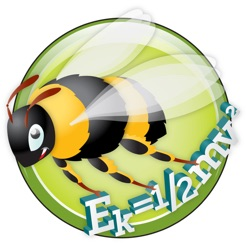## iPad Screenshots

•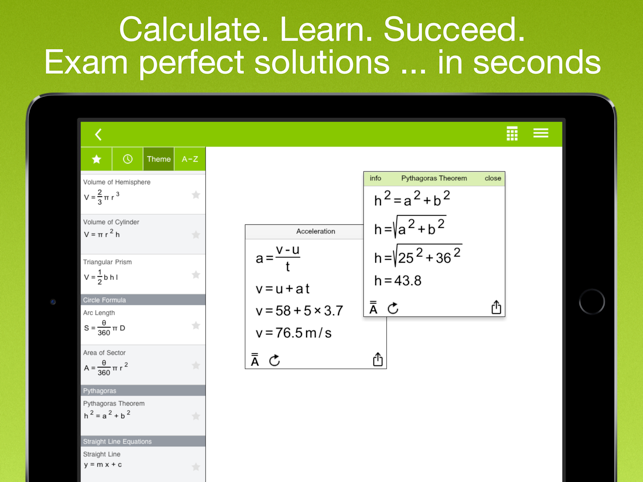•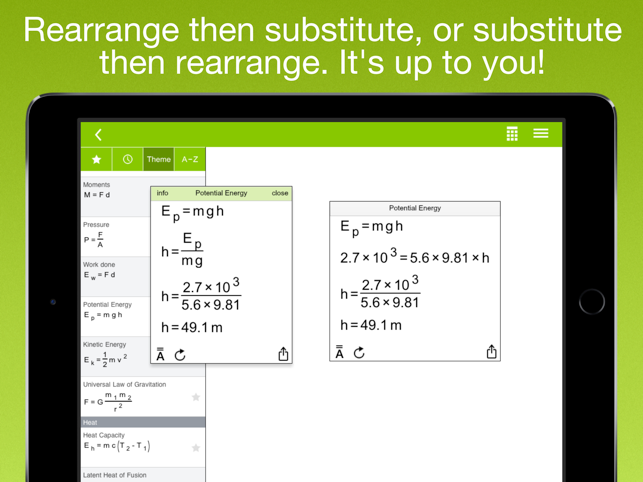•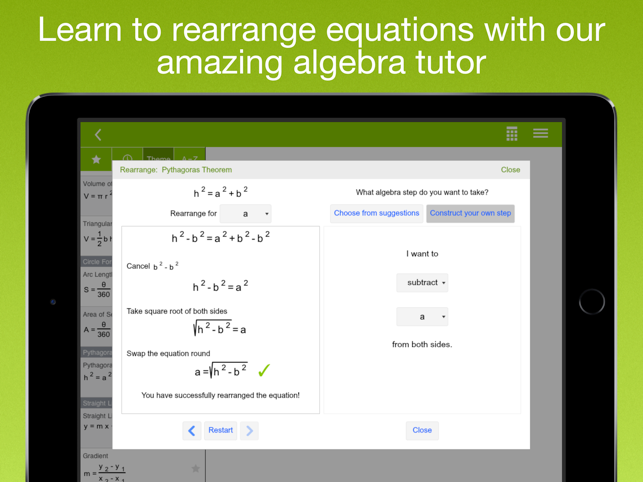•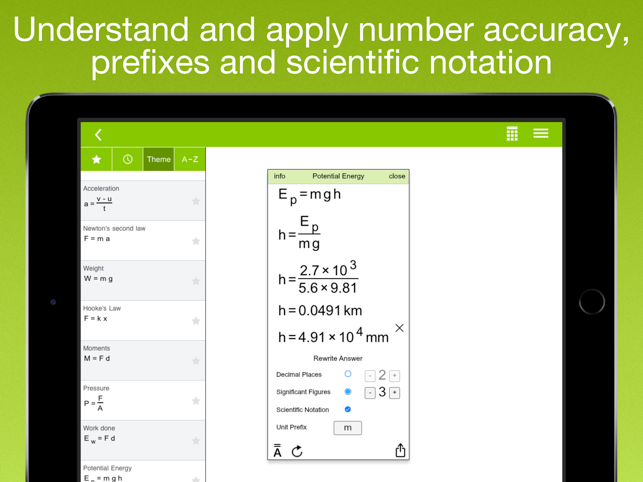•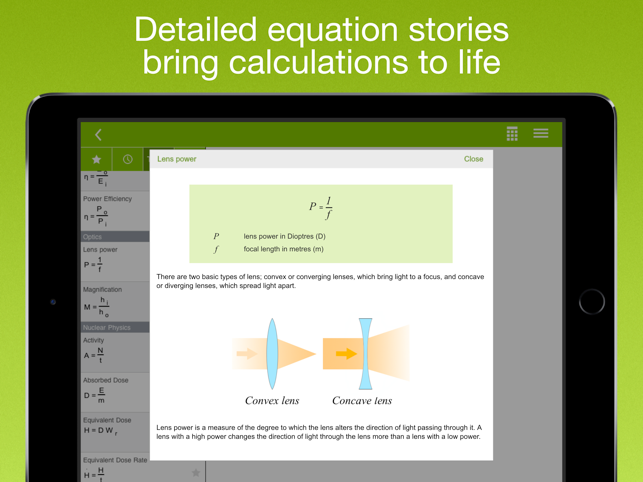•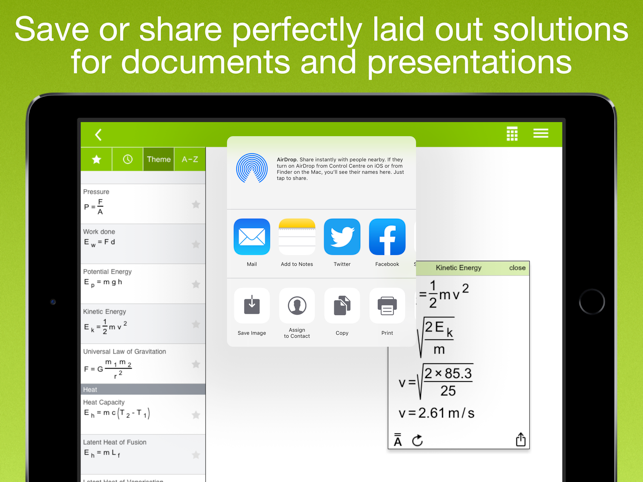•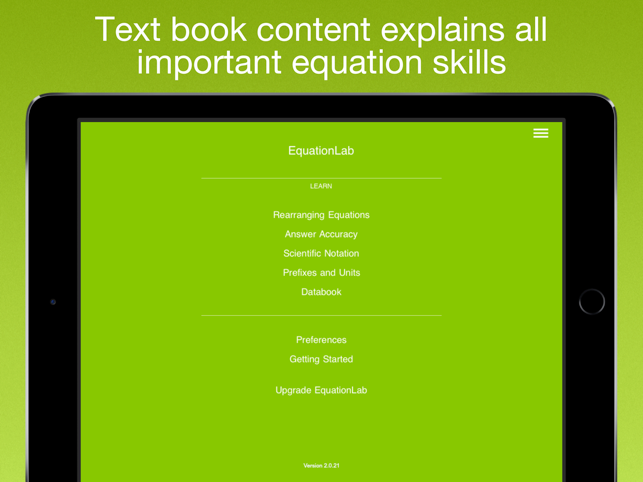•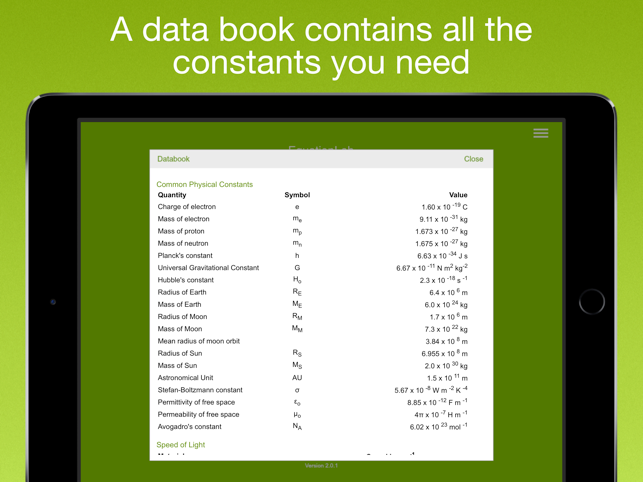•## Description

EquationLab inspires students to calculate, learn equation skills and get great results in High School Physics and Maths. Designed by experienced educators, EquationLab allows students, parents and teachers to do the following:

Students:
• Learn, practise and interact with all the important equation concepts you need to succeed in High School Maths and Physics.
• Easily solve equation based problems for homework and class - everything you need to calculate and learn is in the app.
• Model your written work on EquationLab's solutions.
• Share your calculations with friends on social media.

Parents:
• Check your child's homework is correct without having to remember how to do formula calculations.
• Give your child a resource to support and enrich learning at home.
• Help your child engage with maths and physics in a way which is fun and interactive.

Teachers:
• Enrich classwork and engage students with a ready made formula calculation resource.
• Save time and boost your productivity by creating perfectly laid out calculation resources for documents and presentations.

** EquationLab **

EquationLab enriches a wide range of learning experiences where formula calculation is the focus, encouraging students to engage with this important area. It reinforces an effective calculation process and helps students become secure in their calculation skills. It's also a great productivity tool which saves valuable time when producing equation resources for documents and presentations.

Fast, intuitive drag and drop solving makes calculation easy:
• Select an equation, drag and drop to re-arrange, tap in values and get the answer - it's easy to solve any formula based calculation.
• Get solutions in a fraction of the time it takes with pen, paper and calculator.
• Rearrange your equation and enter values, or enter values in equations first and then rearrange, it's up to you. The latter approach is favoured by some examination boards. EquationLab lets you work both ways.

Interactive algebra tutor:
• Learning to rearrange equations can be a significant hurdle for many young people. Practising rearranging equations using EquationLab's powerful algebra tutor is a fun and engaging way for students to learn.

Perfectly laid out calculations - in seconds:
• Create perfect solutions and easily drop into documents or presentations.
• Save calculation graphics to your iPad, export to other applications, or share on social media with friends and colleagues.

Rich, colourful equation stories:
• Equation explanations and sample calculations show what each physics and maths equation is used for so you can learn while you calculate.

Prefixes, decimal places, significant figures and scientific notation:
• Interact and understand these important concepts as you alter the way your input values and answers are displayed.

Virtual text book content covers all important equation concepts:
• Read about rearranging equations, prefixes, decimal places, significant figures and scientific notation.
• An in app data book lets you quickly look up all important constants.

Scientific calculator:
• Students will need a calculator in exams, so the app has one.

** In App Purchase **

EquationLab can be downloaded free with 6 sample equations. An In App Purchase gives you the full version of the app which contains almost 100 equations, equation stories and unlocks EquationLab's powerful interactive algebra tutor. Having completed the IAP, you will automatically get all future upgrades.

The full equation set includes:

• Maths: Fractions, percentages, circumferences and perimeters, areas, volumes, circle arc and sector, Pythagoras, line and gradient, trigonometry, sine and cosine rule, trigonometric area, roots of a quadratic equation.

• Physics: Mechanics, heat, waves, electricity, power and efficiency, optics, nuclear physics, gas laws and rotational motion.

## What’s New

Version 2.2.4

- Bug fixes
- Performance improvements

## Information

Seller
Go Think Learning Limited
Size
67.6 MB
Category
Education
Compatibility

Requires iOS 8.0 or later. Compatible with iPad.

Languages

English, Czech, Danish, Dutch, Finnish, French, German, Greek, Hebrew, Indonesian, Italian, Japanese, Korean, Malay, Norwegian Bokmål, Polish, Portuguese, Russian, Simplified Chinese, Spanish, Swedish, Thai, Traditional Chinese, Turkish, Vietnamese

Age Rating
Rated 4+
Copyright
© 2015, Go Think Learning Ltd
Price
Free
In-App Purchases
1. Physics and Maths equation set \$7.99

## Supports

•